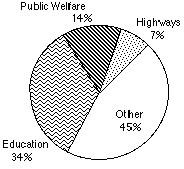## Questions On Quantitative Techniques SMQTQ016Direction for questions 1 – 5: Based on the circle graph given below, answer the following questions.

Expenditure for State and Local Governments1. What type of information is being presented on this graph?

a. Expenditure for education
b. Expenditure for public welfare
c. Expenditure for state and local government
d. Expenditure for highways

2. If the total spending is \$50,000, how much money was spent on highways?

a. \$3,500
b. \$22,500
c. \$ 15,000
d. \$ 20,000

3. Approximately how many times the amount spending on highways is spent on education?

a.10
b.3
c.5
d.15

4. Approximately what fraction of the total expenditures are spent on highways and public welfare combined?

a. 2/5
b. 1/5
c.1/2
d. 2/3

5. How much money was spent as other expenses

a. 22,500
b. 15,000
c. 30,000
d. 20,000

1. Option – c
The graph title is Expenditures for State and Local Governments. The circle is cut into four sectors, each representing one category of expenditures, with the percentage of the total for each category.

2. Option – a
Money spent on highways is \$3,500 (50,000* 7 /100 ) = \$ 3,500

3. Option – c
State and local governments spent approximately five times more on education than on highways (34%).

4. Option – b
Approximately 1/5 of the total is spent on highways and welfare combined.

5. Option – a
(7 * 50,000/100 + 14 * 50, 000/100 = 3,500 + 7, 000 = 10,500 ) 45 *50,000/100 = 22,500